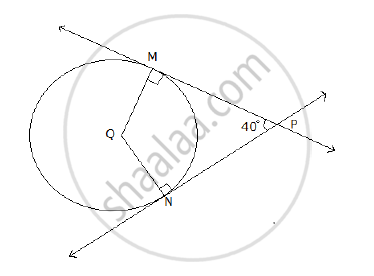SSC (Marathi Semi-English) 10thMaharashtra State Board
Share

# In the following figure, Q is the center of the circle. PM and PN are tangents to the circle. If ∠MPN = 40° , find ∠MQN. - SSC (Marathi Semi-English) 10th - Geometry

ConceptNumber of Tangents from a Point on a Circle

#### Question

In the following figure, Q is the center of the circle. PM and PN are tangents to the circle. If ∠MPN = 40° , find ∠MQN.#### Solution

Given: ∠MPN = 40°

The line perpendicular to a radius of a circle at its outer end is a tangent to the circle.

∠PMQ = 90°       and       ∠QNP = 90°

The sum of the measures of the angles of a quadrilateral is 360°.

∠MPN + ∠PMQ + ∠QNP + ∠MQN = 360°

40° + 90° + 90° + ∠MQN = 360°

220° + ∠MQN = 360°

∠MQN = 360° - 220°

∠MQN = 140°

Is there an error in this question or solution?

#### APPEARS IN

2013-2014 (March) (with solutions)
Question 2.2 | 2.00 marks
Solution In the following figure, Q is the center of the circle. PM and PN are tangents to the circle. If ∠MPN = 40° , find ∠MQN. Concept: Number of Tangents from a Point on a Circle.
S